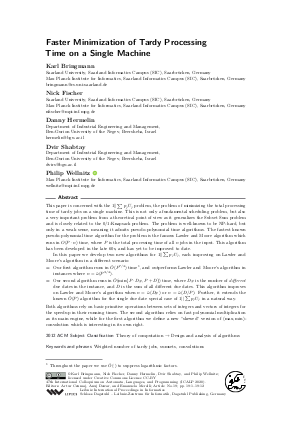Document# Faster Minimization of Tardy Processing Time on a Single Machine

### Authors Karl Bringmann, Nick Fischer, Danny Hermelin, Dvir Shabtay, Philip Wellnitz## File

LIPIcs.ICALP.2020.19.pdf
• Filesize: 496 kB
• 12 pages

## Cite As

Karl Bringmann, Nick Fischer, Danny Hermelin, Dvir Shabtay, and Philip Wellnitz. Faster Minimization of Tardy Processing Time on a Single Machine. In 47th International Colloquium on Automata, Languages, and Programming (ICALP 2020). Leibniz International Proceedings in Informatics (LIPIcs), Volume 168, pp. 19:1-19:12, Schloss Dagstuhl - Leibniz-Zentrum für Informatik (2020)
https://doi.org/10.4230/LIPIcs.ICALP.2020.19

## Abstract

This paper is concerned with the 1||∑ p_jU_j problem, the problem of minimizing the total processing time of tardy jobs on a single machine. This is not only a fundamental scheduling problem, but also a very important problem from a theoretical point of view as it generalizes the Subset Sum problem and is closely related to the 0/1-Knapsack problem. The problem is well-known to be NP-hard, but only in a weak sense, meaning it admits pseudo-polynomial time algorithms. The fastest known pseudo-polynomial time algorithm for the problem is the famous Lawler and Moore algorithm which runs in O(P ⋅ n) time, where P is the total processing time of all n jobs in the input. This algorithm has been developed in the late 60s, and has yet to be improved to date. In this paper we develop two new algorithms for 1||∑ p_jU_j, each improving on Lawler and Moore’s algorithm in a different scenario: - Our first algorithm runs in Õ(P^{7/4}) time, and outperforms Lawler and Moore’s algorithm in instances where n = ω̃(P^{3/4}). - Our second algorithm runs in Õ(min{P ⋅ D_#, P + D}) time, where D_# is the number of different due dates in the instance, and D is the sum of all different due dates. This algorithm improves on Lawler and Moore’s algorithm when n = ω̃(D_#) or n = ω̃(D/P). Further, it extends the known Õ(P) algorithm for the single due date special case of 1||∑ p_jU_j in a natural way. Both algorithms rely on basic primitive operations between sets of integers and vectors of integers for the speedup in their running times. The second algorithm relies on fast polynomial multiplication as its main engine, while for the first algorithm we define a new "skewed" version of (max,min)-convolution which is interesting in its own right.

## Subject Classification

##### ACM Subject Classification
• Theory of computation → Design and analysis of algorithms
##### Keywords
• Weighted number of tardy jobs
• sumsets
• convolutions

## Metrics

• Access Statistics
• Total Accesses (updated on a weekly basis)
0

## References

1. Amir Abboud, Karl Bringmann, Danny Hermelin, and Dvir Shabtay. SETH-based lower bounds for subset sum and bicriteria path. Proc. of of the 30th Annual ACM-SIAM Symposium on Discrete Algorithms (SODA), pages 41-57, 2019.2. Karl Bringmann. A near-linear pseudopolynomial time algorithm for subset sum. In Proc. of of the 28th Annual ACM-SIAM Symposium on Discrete Algorithms (SODA), pages 1073-1084, 2017.3. Thomas H. Cormen, Charles E. Leiserson, Ronald L. Rivest, and Clifford Stein. Introduction to Algorithms, Third Edition. The MIT Press, 3rd edition, 2009.4. Marek Cygan, Marcin Mucha, Karol Wegrzycki, and Michal Wlodarczyk. On problems equivalent to (min, +)-convolution. ACM Trans. Algorithms, 15(1):14:1-14:25, 2019.5. Ronald L. Graham. Bounds on multiprocessing timing anomalies. SIAM Journal on Applied Mathematics, 17(2):416-429, 1969.6. Richard M. Karp. Reducibility among combinatorial problems. In Complexity of computer computations, pages 85-103. Springer, 1972.7. Konstantinos Koiliaris and Chao Xu. A faster pseudopolynomial time algorithm for subset sum. In Proc. of the 28th Annual ACM-SIAM Symposium on Discrete Algorithms (SODA), pages 1062-1072, 2017.8. Sambasiva R. Kosaraju. Efficient tree pattern matching. In Proc. of the 30th annual symposium on Foundations Of Computer Science (FOCS), pages 178-183, 1989.9. Marvin Künnemann, Ramamohan Paturi, and Stefan Schneider. On the fine-grained complexity of one-dimensional dynamic programming. In Proc. of the 44th International Colloquium on Automata, Languages, and Programming (ICALP), pages 21:1-21:15, 2017.10. Eugene L. Lawler and James M. Moore. A functional equation and its application to resource allocation and sequencing problems. Management Science, 16(1):77-84, 1969.X

Feedback for Dagstuhl Publishing